# R: Matrix Operations

Imagine the following problem: You have a business assembling cabinets. You have 10 employees and you want to see who is the most efficient. You track the number of cabinets assembled by each employee over 10 days. You also track the hours they worked each day in a separate table.

Let’s create our tables –

Number of Cabinets

Create a vector of 100 random data points from 50 to 100.

```# created random set of 100 numbers between 50 and 100
x <- sample(50:100,100, T)
x```

sample(range,number of data, allow repeat of data (T,F))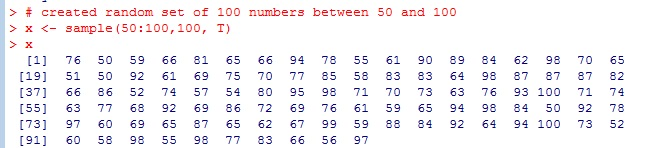Convert to a matrix

```# convert to a 10x10 matrix
A <- matrix(x,10,10)
A```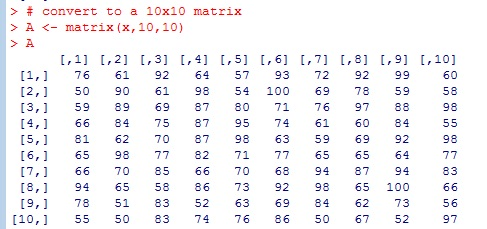Name Columns and Rows

```#name rows and columns
colnames(A) <- c("D1","D2","D3","D4","D5","D6","D7","D8","D9","D10")
row.names(A) <- c("Bob","Steve","Gary","Sara","Tony","Stacey","Kerri","Debbie","George","Manny")
A```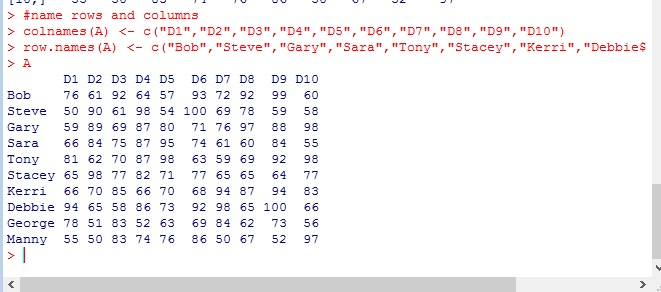Hours Worked

Let’s do the same thing, but now build our hours table.

```# created random set of 100 numbers between 1 and 9
y <- sample(1:9,100, T)
y```Convert to 10×10 matrix form

```# convert to a 10x10 matrix
B <- matrix(y,10,10)
B```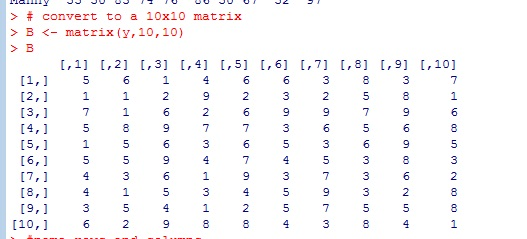Name our columns and rows

```#name rows and columns
colnames(B) <- c("D1","D2","D3","D4","D5","D6","D7","D8","D9","D10")
row.names(B) <- c("Bob","Steve","Gary","Sara","Tony","Stacey","Kerri","Debbie","George","Manny")
B```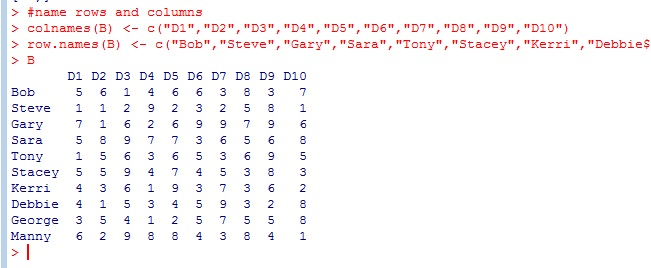## Matrix Operations

Now we will see the magic of R. This programming language was built for Statisticians. So it has some great matrix operations that make linear algebra operations a breeze. In fact, if you ever took linear algebra, you will wish you had known about R back then.

Now if I want to know how many cabinets each person made per hour worked on any given day, I would need to divide each elements of matrix A by its corresponding element in matrix B.

Taking what you know about For loops, think for a minute what the loop would look like to complete this task. Messy huh?

Well in R, we don’t have to worry it. Because in a R, A/B does just what we are asking for.

```#matrix division
C = A/B
C```The results are bit much, let’s round it to 1 decimal place

```#round Matrix
round(C, digits =1)```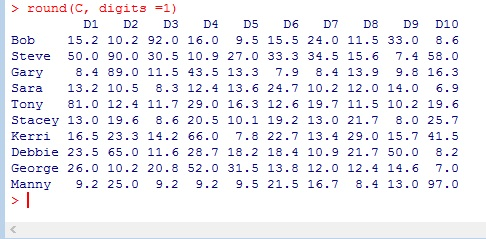Okay, now let’s find the most and least productive values

```#most and least
max(C)
min(C)```

So we have one person who made 97 cabinets in an hour and another who made 6.875Who are they? And what day was it?

```#who are they?
which(C==max(C), arr.ind=T)
which(C==min(C), arr.ind=T)```

Manny is our hero on day 10, and Sara must have been hung over on day 10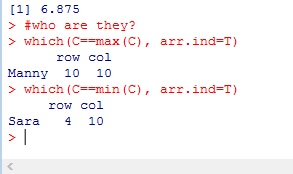I know, I know, if you are here, then you like me are a stat geek. So let’s run the mean and see who is the overall Rockstar.

```#get the means
colMeans(C)
rowMeans(C)```

I have underlined highest and lowest, both day and employee. Looks like Sara is a real slacker.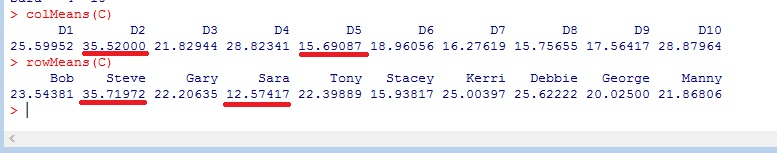Now before you start sending hate mail and calling me sexist, remember these numbers were randomly generated. If I ran my code again, I would get completely different answers. Just as if you follow along, your answers will not match up with mine.

## The Code

```# created random set of 100 numbers between 50 and 100
x <- sample(50:100,100, T)
x
# convert to a 10x10 matrix
A <- matrix(x,10,10)
A
#name rows and columns
colnames(A) <- c("D1","D2","D3","D4","D5","D6","D7","D8","D9","D10")
row.names(A) <- c("Bob","Steve","Gary","Sara","Tony","Stacey","Kerri","Debbie","George","Manny")
A

# created random set of 100 numbers between 1 and 9
y <- sample(1:9,100, T)
y
# convert to a 10x10 matrix
B <- matrix(y,10,10)
B
#name rows and columns
colnames(B) <- c("D1","D2","D3","D4","D5","D6","D7","D8","D9","D10")
row.names(B) <- c("Bob","Steve","Gary","Sara","Tony","Stacey","Kerri","Debbie","George","Manny")
B

#matrix division
C = A/B
C

#round Matrix
round(C, digits =1)

#most and least productive values
max(C)
min(C)

#who are they?
which(C==max(C), arr.ind=T)
which(C==min(C), arr.ind=T)

#get the means
colMeans(C)
rowMeans(C)```

## 3 thoughts on “R: Matrix Operations”

1.Anonymous

I enjoyed the post Ben. I wanted to see if you could clear up one point for me. You make the statement that the numbers are randomly generated and that each run would return a different set of numbers. Is that based on the fact that the data being read in with each pass changes, meaning one person may speed up production, while another may slow down, thus changing the values being calculated? I ask this because it seems in a case such as this you would not want a random value returned but you would want more consistency in the results. If I have X number of employees producing X number of goods, as a business owner I would expect to see a certain amount of consistency in the productivity numbers amongst my employees. Just an initial though I had. Thanks for the great content.

1.Ben Larson

Thanks for visiting my site and taking the time to reply. As to your question, when I talk about my randomly generated numbers, I am referring to the way I created the matrices in this lesson. In a real world case, yes I would want actual real non-randomly created data, but in this lesson I wanted to show you how easy R makes it for you to create your own practice data sets. Using sample(50:100,100,T) function, I am able to create a vector with 100 elements between 50 and 100 in one quick line of code.

So when I say each time I run it, I will get a new set of data, it am referring to the two sample() functions I run at the beginning of my code to create my two matrices.

2.Kiersten

Hey just wanted to give you a quick heads
up. The words in your article seem to be running off the screen in Safari.
I’m not sure if this is a format issue or something to do with web browser compatibility but I figured
I’d post to let you know. The design look great though!
Hope you get the issue solved soon. Many thanks